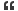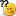1. ## c#

what will be the o/p of following?
int x=10;
Console.Writeline("(2)(1)(0)",++x,x++,x++);Reply With Quote

2. ## Re: c#

what will be the o/p of following?
int x=10;
Console.Writeline("(2)(1)(0)",++x,x++,x++);Reply With Quote

3. ## Re: c#Reply With Quote

4. ## Re: c#

It should be 11,10,10

as first time is goes to ++x, x will become 11
and when it goes to x++, it will remain 10, as the value of x is 10Reply With Quote

5. ## Re: c#

execution starts from right side....x++....10.... x becomes 11
x++....11.... x becomes 12
++x...pre increment i,e x becomes 13
output is 10,11,13Reply With Quote

6. ## Re: c#

the answear is 11Reply With Quote

7. ## Re: c#

it should be 10 , 11 , 13Reply With Quote

8. ## Re: c#Originally Posted by SOMRAJwhat will be the o/p of following?
int x=10;
Console.Writeline("(2)(1)(0)",++x,x++,x++);Reply With Quote

9. ## Re: c#

the answer is 10 11 13Reply With Quote

10. ## Re: c#Originally Posted by SOMRAJwhat will be the o/p of following?
int x=10;
Console.Writeline("(2)(1)(0)",++x,x++,x++);Reply With Quote

11. ## Re: c#Originally Posted by SOMRAJwhat will be the o/p of following?
int x=10;
Console.Writeline("(2)(1)(0)",++x,x++,x++);Reply With Quote

12. ## Re: c#

11 11 12 will be the answer...

of ++x,x++,x++ consider as
1 2 3 x's

for 1 the x value will be increased to 11 and is displayed
for 2 the x value is first displayed as 11 and then increased to 12
for 3 the x value is first displayed as 12 and then increased to 13

To Test... in C#
int x = 10;
Response.Write(++x + "--" + x++ + "--" + x++);
Response.Write("At last X value is "+x);

11--11--12 At last X value is 13Reply With Quote

13. ## Re: c#

it Should be 11,11,12 and in last x shuld be equal to 13Reply With Quote

14. ## Re: c#

NothingBecause there is no such function Writeline , you have to use WriteLineIf you use WriteLine , you will receive
(2)(1)(0)Reply With Quote

15. ## Re: c#

The correct ans. is 10,11,13....
Bcoz first time it goes on 2nd index means x++, so first it assign or print a value thai is 10.
after that it increment x value by 1 so now x = 11,
then again it print 11 and then it incremented so x= 12,
then first it increment so x=13 and it print.

so, final ans. is 10,11,13.Reply With Quote

16. ## Re: c#

Err, its (2)(1)(0) because there are not any placeholders.

It should be {2}{1}{0}.

Which results in 12,11,11.

First operation = 11;
Second Operation = 12; but doesn't get incremented until the operation completes so output is 11.
Third Operation = 13; but current value is 12.Reply With Quote

17.

####Posting Permissions

• You may not post new threads
• You may not post replies
• You may not post attachments
• You may not edit your posts
•